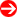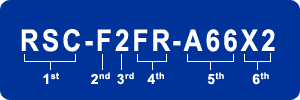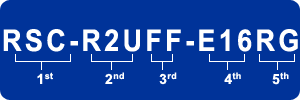#Product Naming Convention - Riser CardsCharacter (Set) Representation Options 1st Part Number Prefix • RSC = Riser Card 2nd Product Category • S = Standard         • U = Ultra         • W = WIO         • G = GPU         • T = Twin         • F = FatTwin         • P = TwinPro         • M = MicroBlade         • B = SuperBlade         • C = MicroCloud         • X = Storage         • D = DCO 3rd Form Factor • 1 = 1U         • 2 = 2U         • 3 = 3U         • 4 = 4U         • 5 = 5U 4th Location • R = Right hand side         • F = Front IO/Front of the MB         • B = Rear IO/Front of the MB         • M = M.2 Connector         • N = NVMe Connector 5th Output • A = Active Riser Card         • U = UIO         • 4/8/6 = PCI-E x4/x8/x16         • X = PCI-X         • X33 = PCI 6th MB Type/Spec • PR = Proprietary         • + = Additional Features         • E = MB with Spec         • H = Support AMD MB only         • UP = Support UP MB         • X = Support X10 MB & above         • X1 = Support X11 MB & above         • X2 = Support X12 MB & above         • QP = Support 4-way system         • OP = Support 8-way system         • Gx = PCI-E Gen-x support (x: 3, 4, 5)

#Product Naming Convention - Riser Cards (previous generation)Character Set Representation Options 1st Part Number Prefix • RSC = Riser Card (New Naming Conventions)         • CSE = Accessory Prefix (Old Naming Conventions) 2nd Riser Card Type • R1U = 1U Riser         • RR1U = 1U Riser         • R2U = 2U Riser (New Naming Conventions)         • RR2U = 2U Riser (Old Naming Conventions) 3rd I/O Slot Type (Connect to Motherboard) • U = Fits UIO MB         • W = Fits WIO MB         • G = Fits GPU MBs         • T = Fits Twin MB         • F = Fits FatTwin MBs         • FF = Fits FatTwin MBs w/ Front I/O         • FR = Fits FatTwin MBs w/ Rear I/O         • EP = Fits MB w/ SEPC (Supermicro PCI-E Power Connector)         • XE = Fits MB w/ SXB-E (Super Extended Bus, 128bit PCI slot) 4th I/O Slots on Riser • U = UIO Slot         • E4 = 1 PCI-E x4 Slot         • E8 = 1 PCI-E x8 Slot         • E16 = 1 PCI-E x16 Slot         • X = PCI-X Slot         • X33 = PCI Slot         • A = Active 5th I/O Slots on Riser • + = Additional Feature (such as Gen2 support)         • B = Riser Card Fits in Rear side of the MB         • A = Riser Card Fits in Front side of the MB         • L = Left Side Riser         • R = Right Side Riser         • G = GPU Cards         • PR = Proprietary         • X9 = Supported on X9 Platform         • UP = Support Single Processor Motherboard